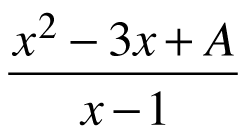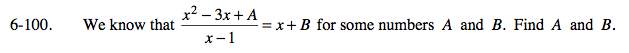### Home > PC > Chapter 6 > Lesson 6.2.3 > Problem6-100

6-100.

We know that= x + B for some numbers A and B. Find A and B. Homework Help ✎Multiply both sides by (x − 1).

x2 − 3x + A = (x + B)(x − 1)

Multiply out on the right side.

x2 − 3x + A = x2 + BxxB

Subtract x2 from both sides.

−3x + A = BxxB

Subtract x from both sides of the equation.

−2x + A = BxB

Set the x-terms = to each other.
Set the constant terms = to each other.

∴ − 2 = B and A = − B
A = 2